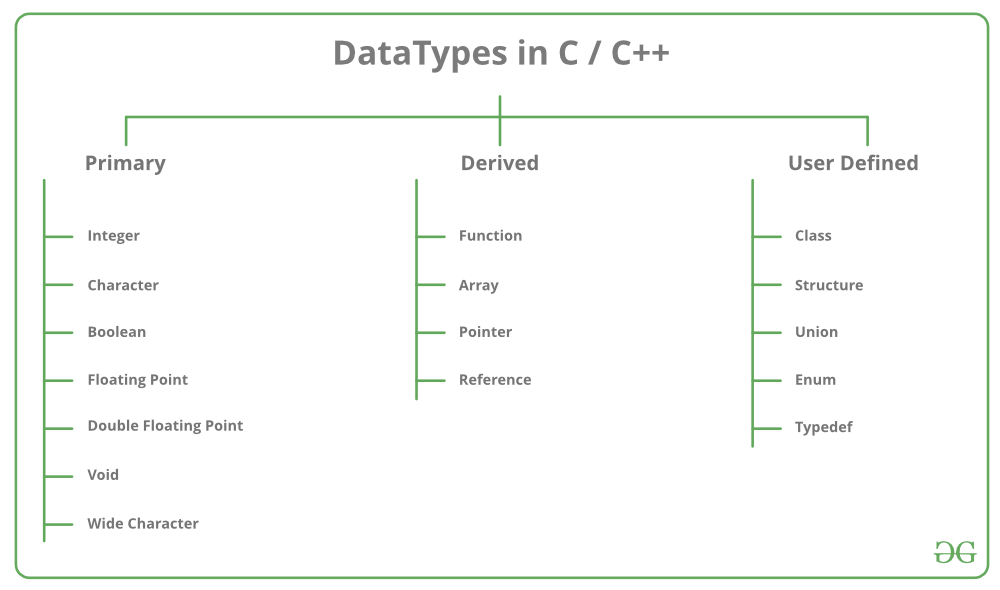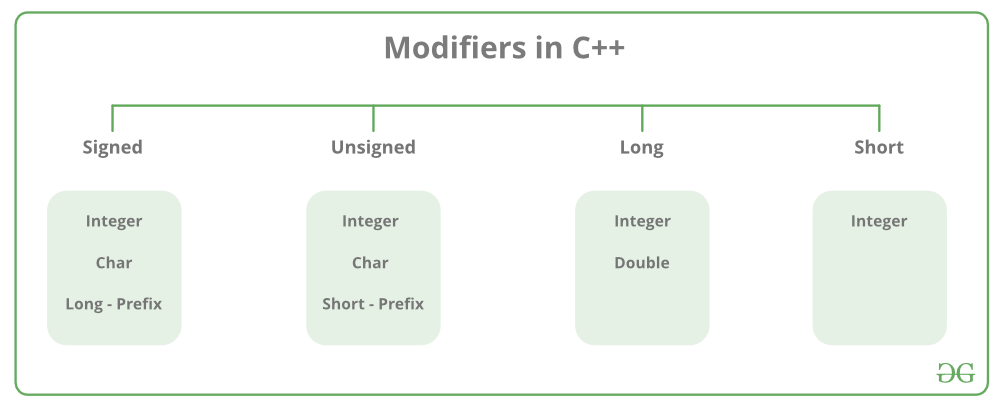GFG App
Open AppBrowser
Continue

# C++ Data Types

All variables use data type during declaration to restrict the type of data to be stored. Therefore, we can say that data types are used to tell the variables the type of data they can store. Whenever a variable is defined in C++, the compiler allocates some memory for that variable based on the data type with which it is declared. Every data type requires a different amount of memory.

C++ supports a wide variety of data types and the programmer can select the data type appropriate to the needs of the application. Data types specify the size and types of values to be stored. However, storage representation and machine instructions to manipulate each data type differ from machine to machine, although C++ instructions are identical on all machines.

C++ supports the following data types:

1. Primary or Built-in or Fundamental data type
2. Derived data types
3. User-defined data types## Data Types in C++ are Mainly Divided into 3 Types:

1. Primitive Data Types: These data types are built-in or predefined data types and can be used directly by the user to declare variables. example: int, char, float, bool, etc. Primitive data types available in C++ are:

• Integer
• Character
• Boolean
• Floating Point
• Double Floating Point
• Valueless or Void
• Wide Character

2. Derived Data Types: Derived data types that are derived from the primitive or built-in datatypes are referred to as Derived Data Types. These can be of four types namely:

• Function
• Array
• Pointer
• Reference

3. Abstract or User-Defined Data Types: Abstract or User-Defined data types are defined by the user itself. Like, defining a class in C++ or a structure. C++ provides the following user-defined datatypes:

• Class
• Structure
• Union
• Enumeration
• Typedef defined Datatype

## Primitive Data Types

• Integer: The keyword used for integer data types is int. Integers typically require 4 bytes of memory space and range from -2147483648 to 2147483647.
• Character: Character data type is used for storing characters. The keyword used for the character data type is char. Characters typically require 1 byte of memory space and range from -128 to 127 or 0 to 255.
• Boolean: Boolean data type is used for storing Boolean or logical values. A Boolean variable can store either true or false. The keyword used for the Boolean data type is bool
• Floating Point: Floating Point data type is used for storing single-precision floating-point values or decimal values. The keyword used for the floating-point data type is float. Float variables typically require 4 bytes of memory space.
• Double Floating Point: Double Floating Point data type is used for storing double-precision floating-point values or decimal values. The keyword used for the double floating-point data type is double. Double variables typically require 8 bytes of memory space.
• void: Void means without any value. void data type represents a valueless entity. A void data type is used for those function which does not return a value.
• Wide Character: Wide character data type is also a character data type but this data type has a size greater than the normal 8-bit data type. Represented by wchar_t. It is generally 2 or 4 bytes long.
• sizeof() operator: sizeof() operator is used to find the number of bytes occupied by a variable/data type in computer memory.

Example:

int m , x;

cout<<sizeof(m); //returns 4 which is the number of bytes occupied by the integer variable “m”.

cout<<sizeof(x); //returns 200 which is the number of bytes occupied by the integer array variable “x”.

The size of variables might be different from those shown in the above table, depending on the compiler and the computer you are using.

## C++

 `// C++ Program to Demonstrate the correct size` `// of various data types on your computer.` `#include ` `using` `namespace` `std;`   `int` `main()` `{` `    ``cout << ``"Size of char : "` `<< ``sizeof``(``char``) << endl;` `    ``cout << ``"Size of int : "` `<< ``sizeof``(``int``) << endl;`   `    ``cout << ``"Size of long : "` `<< ``sizeof``(``long``) << endl;` `    ``cout << ``"Size of float : "` `<< ``sizeof``(``float``) << endl;`   `    ``cout << ``"Size of double : "` `<< ``sizeof``(``double``) << endl;`   `    ``return` `0;` `}`

Output

```Size of char : 1
Size of int : 4
Size of long : 8
Size of float : 4
Size of double : 8```

Time Complexity: O(1)

Space Complexity: O(1)

## Datatype Modifiers

As the name suggests, datatype modifiers are used with built-in data types to modify the length of data that a particular data type can hold.Data type modifiers available in C++ are:

• Signed
• Unsigned
• Short
• Long

The below table summarizes the modified size and range of built-in datatypes when combined with the type modifiers:

Note: Above values may vary from compiler to compiler. In the above example, we have considered GCC 32 bit.
We can display the size of all the data types by using the sizeof() operator and passing the keyword of the datatype, as an argument to this function as shown below:

Now to get the range of data types refer to the following chart

Note: syntax<limits.h> header file is defined to find the range of fundamental data-types. Unsigned modifiers have minimum value is zero. So, no macro constants are defined for the unsigned minimum value.

## Macro Constants

The actual value depends on the particular system and library implementation but shall reflect the limits of these types in the target platform. LLONG_MIN, LLONG_MAX, and ULLONG_MAX are defined for libraries complying with the C standard of 1999 or later (which only includes the C++ standard since 2011: C++11).

C++ Program to Find the Range of Data Types using Macro Constants

Example:

## C++

 `// C++ program to Demonstrate the sizes of data types` `#include ` `#include ` `using` `namespace` `std;`   `int` `main()` `{` `    ``cout << ``"Size of char : "` `<< ``sizeof``(``char``) << ``" byte"` `         ``<< endl;`   `    ``cout << ``"char minimum value: "` `<< CHAR_MIN << endl;`   `    ``cout << ``"char maximum value: "` `<< CHAR_MAX << endl;`   `    ``cout << ``"Size of int : "` `<< ``sizeof``(``int``) << ``" bytes"` `         ``<< endl;`   `    ``cout << ``"Size of short int : "` `<< ``sizeof``(``short` `int``)` `         ``<< ``" bytes"` `<< endl;`   `    ``cout << ``"Size of long int : "` `<< ``sizeof``(``long` `int``)` `         ``<< ``" bytes"` `<< endl;`   `    ``cout << ``"Size of signed long int : "` `         ``<< ``sizeof``(``signed` `long` `int``) << ``" bytes"` `<< endl;`   `    ``cout << ``"Size of unsigned long int : "` `         ``<< ``sizeof``(unsigned ``long` `int``) << ``" bytes"` `<< endl;`   `    ``cout << ``"Size of float : "` `<< ``sizeof``(``float``) << ``" bytes"` `         ``<< endl;`   `    ``cout << ``"Size of double : "` `<< ``sizeof``(``double``)` `         ``<< ``" bytes"` `<< endl;`   `    ``cout << ``"Size of wchar_t : "` `<< ``sizeof``(``wchar_t``)` `         ``<< ``" bytes"` `<< endl;`   `    ``return` `0;` `}`

Output

```Size of char : 1 byte
char minimum value: -128
char maximum value: 127
Size of int : 4 bytes
Size of short int : 2 bytes
Size of long int : 8 bytes
Size of signed long int : 8 bytes
Size of unsigned long int : 8 bytes
Size of float : 4 bytes
Size of double : 8 bytes
Size of wchar_t : 4 bytes```

Time Complexity: O(1)

Space Complexity: O(1)

## C++

 `#include ` `#include ` `using` `namespace` `std;`   `int` `main() {` `  ``// Integer data types` `  ``int` `a = 10;` `  ``short` `b = 20;` `  ``long` `c = 30;` `  ``long` `long` `d = 40;` `  ``cout << ``"Integer data types: "` `<< endl;` `  ``cout << ``"int: "` `<< a << endl;` `  ``cout << ``"short: "` `<< b << endl;` `  ``cout << ``"long: "` `<< c << endl;` `  ``cout << ``"long long: "` `<< d << endl;` `  `  `  ``// Floating-point data types` `  ``float` `e = 3.14f;` `  ``double` `f = 3.141592;` `  ``long` `double` `g = 3.14159265358979L;` `  ``cout << ``"Floating-point data types: "` `<< endl;` `  ``cout << ``"float: "` `<< e << endl;` `  ``cout << ``"double: "` `<< f << endl;` `  ``cout << ``"long double: "` `<< g << endl;` `  `  `  ``// Character data types` `  ``char` `h = ``'a'``;` `  ``wchar_t` `i = L``'b'``;` `  ``char16_t j = u``'c'``;` `  ``char32_t k = U``'d'``;` `  ``cout << ``"Character data types: "` `<< endl;` `  ``cout << ``"char: "` `<< h << endl;` `  ``wcout << ``"wchar_t: "` `<< i << endl;` `  ``cout << ``"char16_t: "` `<< j << endl;` `  ``cout << ``"char32_t: "` `<< k << endl;` `  `  `  ``// Boolean data type` `  ``bool` `l = ``true``;` `  ``bool` `m = ``false``;` `  ``cout << ``"Boolean data type: "` `<< endl;` `  ``cout << ``"true: "` `<< l << endl;` `  ``cout << ``"false: "` `<< m << endl;` `  `  `  ``// String data type` `  ``string n = ``"Hello, world!"``;` `  ``cout << ``"String data type: "` `<< endl;` `  ``cout << n << endl;` `  `  `  ``return` `0;` `}`

Output

```Integer data types:
int: 10
short: 20
long: 30
long long: 40
Floating-point data types:
float: 3.14
double: 3.14159
long double: 3.14159
Character data types:
char: a
wchar_t: b
char16_t: 99
char32_t: 100
Boolean data type:
true: 1
false: 0
String data type:
Hello, world!```

This program declares variables of various data types, assigns values to them, and then prints out their values.

The integer data types include int, short, long, and long long. These data types represent whole numbers of varying sizes.

The floating-point data types include float, double, and long double. These data types represent real numbers with varying levels of precision.

The character data types include char, wchar_t, char16_t, and char32_t. These data types represent individual characters of varying sizes.

The boolean data type is a simple data type that can only have one of two values: true or false.

The string data type is a sequence of characters. In this program, we use the string class to declare a string variable and assign it a value.

Data types provide a way to categorize and organize data in a program, making it easier to understand and manage.
Each data type has a specific range of values it can hold, allowing for more precise control over the type of data being stored.
Data types help prevent errors and bugs in a program by enforcing strict rules about how data can be used and manipulated.
C++ provides a wide range of data types, allowing developers to choose the best type for a specific task.